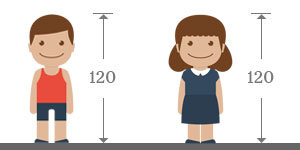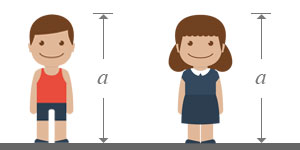# Like algebraic symbols

The algebraic symbols whose representing quantities are same, are called like algebraic symbols.

Quantities are usually denoted by the numbers mathematically but symbols are used instead of the numbers to represent the quantities as per the concept of elementary algebra.

Every symbol represents a particular quantity and different quantities are represented by the different symbols in mathematics. In some cases, two or more quantities are equal. So, the quantities are known as like. In the same way, the algebraic symbols which represent the equal quantities are also like.

Therefore, the algebraic symbols which represent same quantities are called like algebraic symbols algebraically.

## ExampleConsider, the heights of a girl and a boy of same age.

It is measured that the height of a boy is $120$ centimetres and assume it is denoted by a symbol $a$.

Therefore, $a=120$

It is also measured that the height of a girl is also $120$ centimeters and assume it is denoted by a symbol $b$.Therefore, $b=120$

Now, the heights of boy and girl are represented by the two different symbols $a$ and $b$ respectively instead of expressing the heights in numbers.

The height of the girl is equal to the height of the boy. So, the heights of them are like. Therefore, the letter which represents the height of the boy should be equal to the letter which represents the height of the girl.Therefore $a=b$, because $a=120$ and $b=120$

The letter $a$ can be written instead of $b$ and the letter $b$ can be written instead of $a$ due to the like property.

Now, the height of the boy is $a$ and the height of the girl is also $a$. Similarly, the height of the boy is $b$ and the height of the girl is also $b$.

Therefore, the heights of the boy and girl are now $a$ and $a$. Similarly, the heights of the boy and girl are now $b$ and $b$. The letter $a$ is like to $a$. Similarly, the letter $b$ is also like to same symbol algebraically.Due to representing same quantities,

1. The algebraic symbols $a$ and $a$ are called like algebraic symbols.
2. The algebraic symbols $b$ and $b$ are called like algebraic symbols.
3. The algebraic symbols $a$ and $b$ are called as like algebraic symbols in these types of cases only.

If two or more quantities are denoted by only one symbol, or two or more algebraic symbols represent same quantities, they are called like algebraic symbols mathematically.